### Home > CALC > Chapter 5 > Lesson 5.1.4 > Problem5-43

5-43.
1. Using the graph of the slope function f ′(x) below, determine where the following situations occur for f (x): Homework Help ✎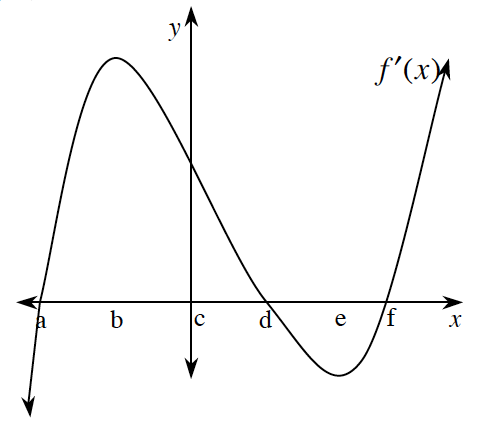1. Relative minima and maxima.

2. Intervals at which f(x) is increasing.

3. Inflection points.

4. Intervals at which f(x) is concave up and concave down.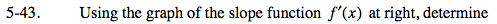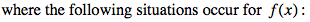Notice that this is a graph of f '(x), but you are being asked about f(x).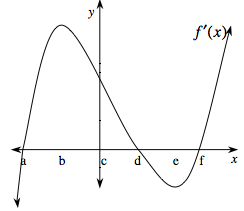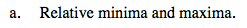Relative minima and maxima can exist when f '(x) = 0. x = a, x = d and x = f. But which indicates a max and which indicates a min?

To distinguish between a relative minimum and a relative maximum, use the 1st or 2nd derivative test.

Refer to the hints in problem 5-42 for more guidance.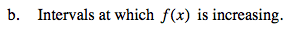f(x) increases when the slope is positive... and we are looking at graph of the slopes.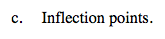Inflection points can happen where f ''(x) = 0... and we are looking at the graph of f '(x).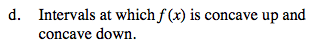f(x) is concave up when f ''(x) > 0... and we are looking at a graph of f '(x).